# 程式分析與優化 - 7 靜態單賦值（SSA）## 7.1 控制流圖回顧

```1 int max(int a, int b) {
2   int ans = a;
3   if (b > a) {
4     ans = b;
5   }
6   return ans;
7 }```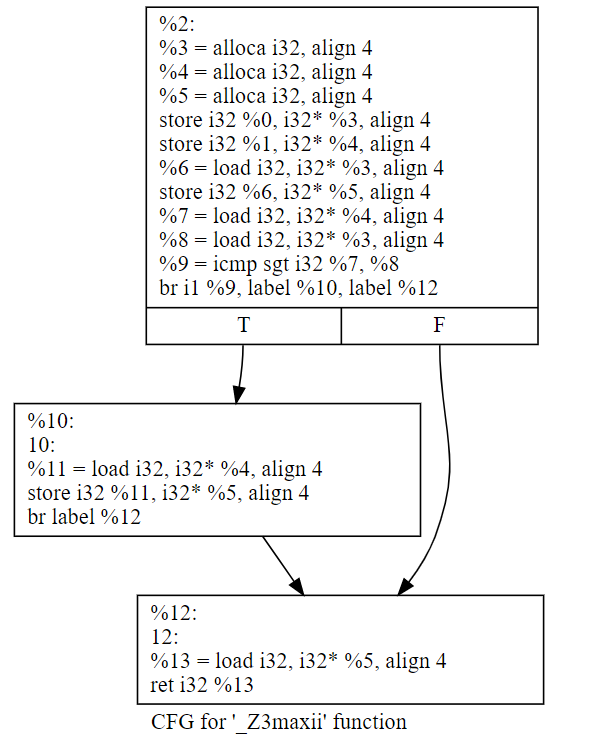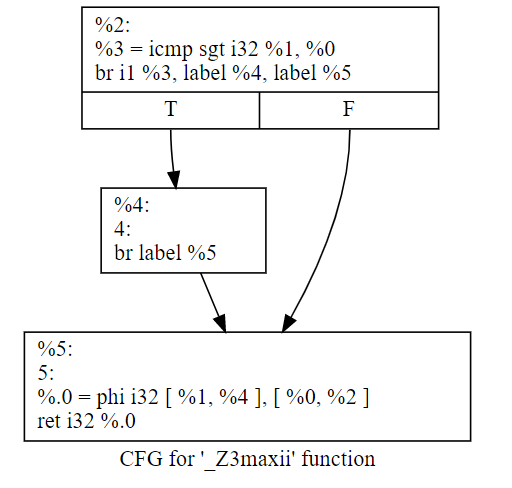除了我們本來準備跑的mem2reg的pass外，優化前後最後一個BB里是不是還多了一個phi函數？

### 7.1.1 靜態單賦值範式（SSA Form）

1991年SSA的奠基論文被引用打到2800+次，這還是截止2019年的數據，這個引用次數每年還在增加。

## 7.2 從SSA來到SSA去

### 7.2.1 將線性代碼轉換成SSA Form

```1 double baskhara(double a, double b, double c) {
2   double delta = b * b - 4 * a * c;
3   double sqrDelta = sqrt(delta);
4   double root = (b + sqrDelta) / 2 * a;
5   return root;
6 }```

``` 1 define double @baskhara(double %a, double %b, double %c) {
2   %1 = fmul double %b, %b
3   %2 = fmul double 4.000000e+00, %a
4   %3 = fmul double %2, %c
5   %4 = fsub double %1, %3
6   %5 = call double @sqrt(double %4)
7   %6 = fadd double %b, %5
8   %7 = fdiv double %6, 2.000000e+00
9   %8 = fmul double %7, %a
10   ret double %8
11 }```

``` 1 for each variable a:
2     Count[a] = 0
3     Stack[a] = 
4 rename_basic_block(B) =
5     for each instruction S in block B:
6         for each use of a variable x in S:
7             i = top(Stack[x])
8             replace the use of x with xi
9         for each variable a that S defines
10             count[a] = Count[a] + 1
11             i = Count[a]
12             push i onto Stack[a]
13             replace definition of a with ai```

```1 a = x + y;
2 b = a - 1;
3 a = y + b;
4 b = 4 * x;
5 a = a + b;```

```1 a1 = x0 + y0;
2 b1 = a1 - 1;
3 a2 = y0 + b1;
4 b2 = 4 * x0;
5 a3 = a2 + b2;```

### 7.2.2 Phi函數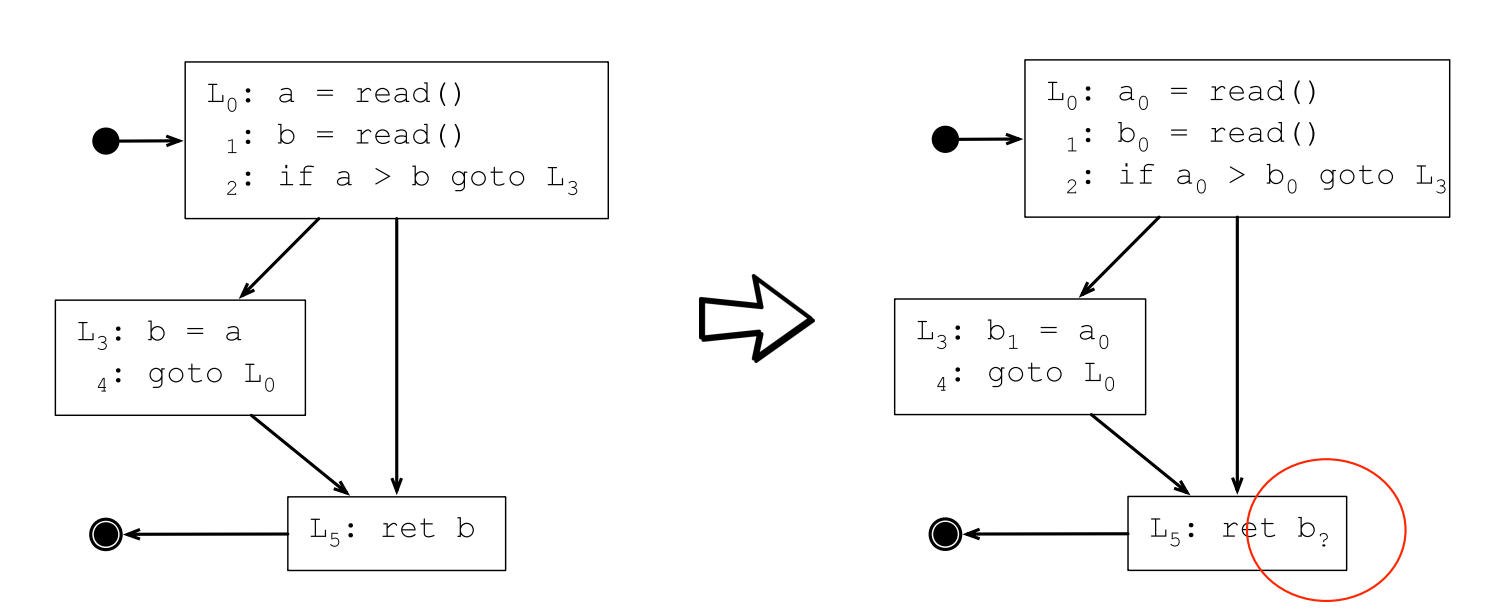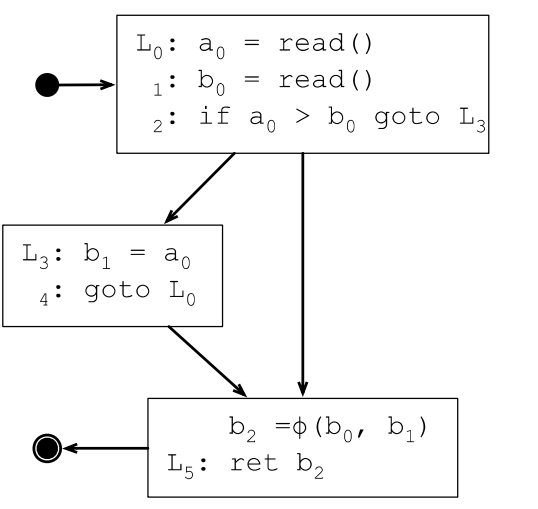φ函數會插入到每個基本塊的最開始地方，對N個變數生成N個φ函數，φ函數的參數個數取決於執行到該基本塊的直接前驅有幾個。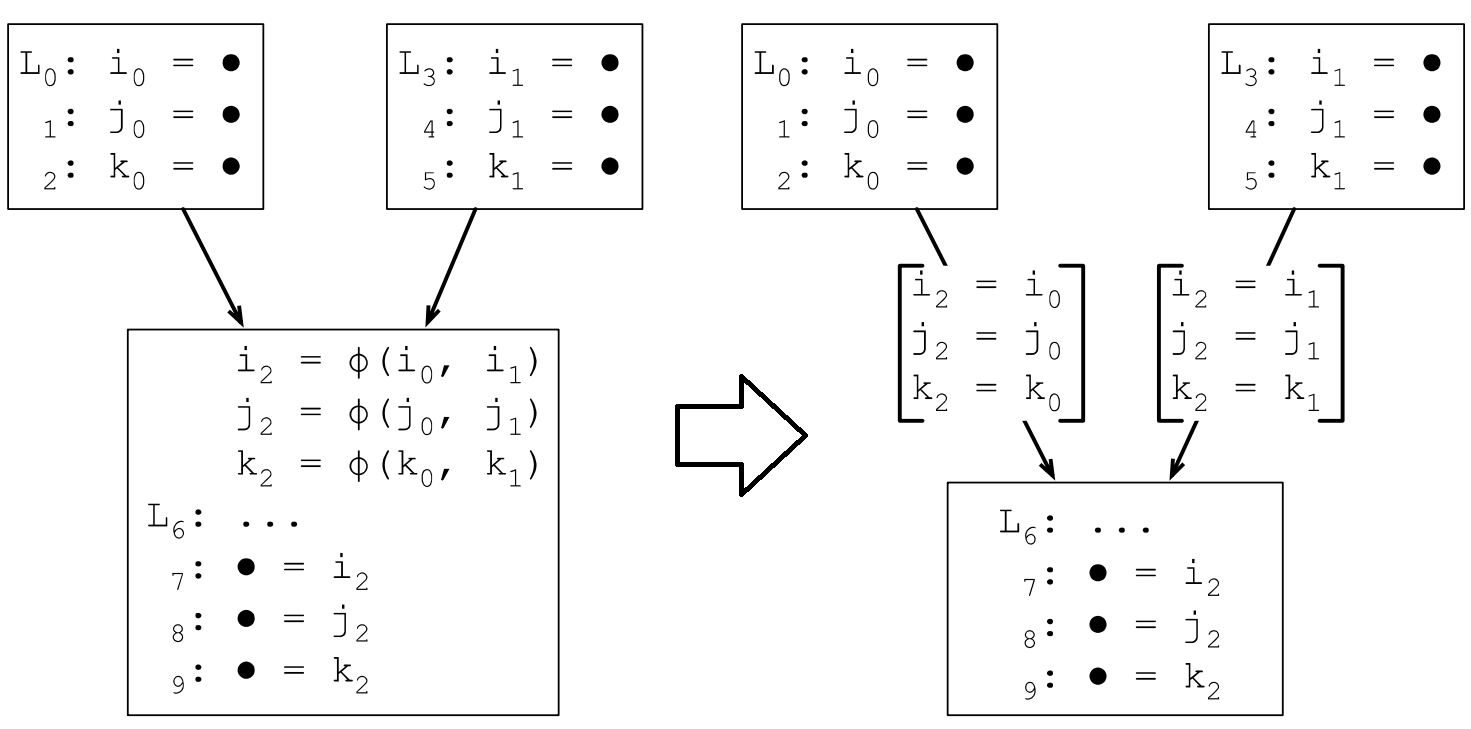### 7.2.5 φ函數的插入策略

• 存在一個基本塊x包含b的定義
• 存在一個非x的基本塊y包含b的定義
• 存在至少一條路徑Pxz從x到z
• 存在至少一條路徑Pyz從y到z
• Pyz和Pxz除了z節點外，沒有其他公共節點
• z不會同時出現在Pxz和Pyz路徑中間，但可以出現在其中一條路徑的中間

### 7.2.8 支配前沿的計算

DF[n] = DFlocal[n] ∪ { DFup[c] | c ∈ children[n] }
Where:
DFlocal[n]: 不被n嚴格支配（SSA的1989年版本要求的是嚴格支配，但1991年版本優化成直接支配，前一篇在ACM會議上，後一篇在ACM期刊上，Cytron果然是混職級的高手）的n的後繼節點
DFup[c]: c的支配前沿集合中被n嚴格支配的節點
children[n]: 支配樹中n的子結點集合

``` 1 computeDF[n]:
2 S = {}
3 for each node y in succ[n]
4     if idom(y) ≠ n
5         S = S ∪ {y}
6 for each child c of n in the dom-tree
7     computeDF[c]
8     for each w ∈ DF[c]
9         if n does not dom w, or n = w
10             S = S ∪ {w}
11 DF[n] = S```

### 7.2.9 插入φ函數

``` 1 place-phi-functions:
2   for each node n:
3     for each variable a ∈ Aorig[n]:
4       defsites[a] = defsites[a] ∪ [n]
5   for each variable a:
6     W = defsites[a]
7     while W ≠ empty list
8       remove some node n from W
9       for each y in DF[n]:
10       if a ∉ Aphi[y]
11         insert-phi(y, a)
12         Aphi[y] = Aphi[y] ∪ {a}
13         if a ∉ Aorig[y]
14         W = W ∪ {y}
15
16 insert-phi(y, a):
17   insert the statement a = ϕ(a, a, …, a)
18   at the top of block y, where the
19   phi-function has as many arguments
20   as y has predecessors
21 Where:
22 Aorig[n]:  the  set  of  variables  defined  at  node  "n"
23 Aphi[y]:  the  set  of  variables  that  have  phi-functions  at  node  "y"```

### 7.2.10 變數重命名

``` 1 rename(n):
2   rename-basic-block(n)
3   for each successor Y of n, where n is the j-th predecessor of Y:
4     for each phi-function f in Y, where the operand of f is ‘a’
5       i = top(Stack[a])
6       replace j-th operand with ai
7   for each child X of n:
8     rename(X)
9   for each instruction S ∈ n:
10     for each variable v that S defines:
11       pop Stack[v]```
`rename-basic-block的定義參照之前的，這裡只是增加了一些場景。`

## 7.3 跑一下整個流程

### 7.3.1 偽代碼

``` 1 i = 1
2 j = 1
3 k = 0
4 while k < 100
5   if j < 20
6     j = i
7     k = k + 1
8   else
9     j = k
10     k = k + 2
11 return j```

### 7.3.2 生成控制流圖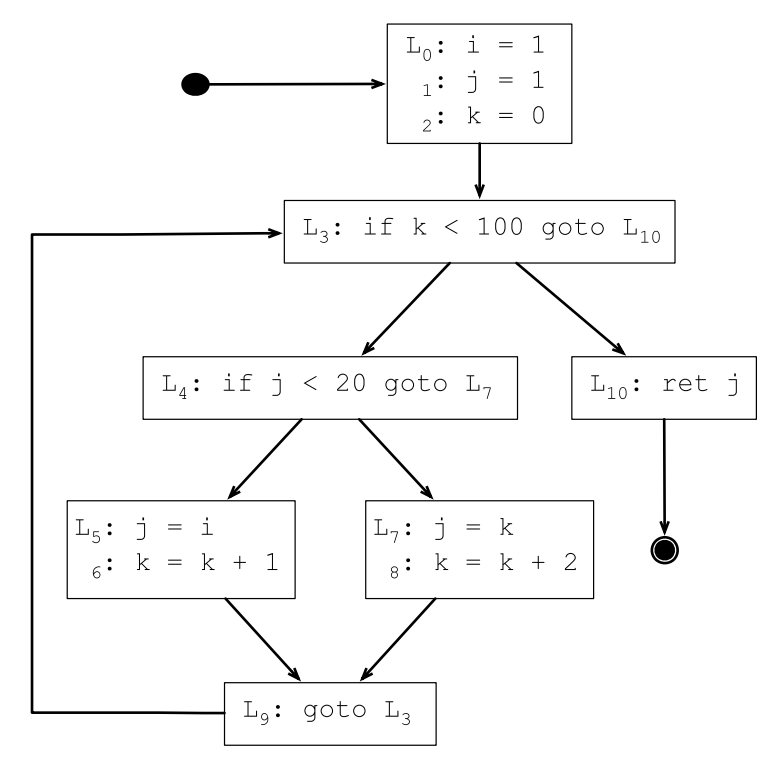### 7.3.3 根據控制流圖生成支配樹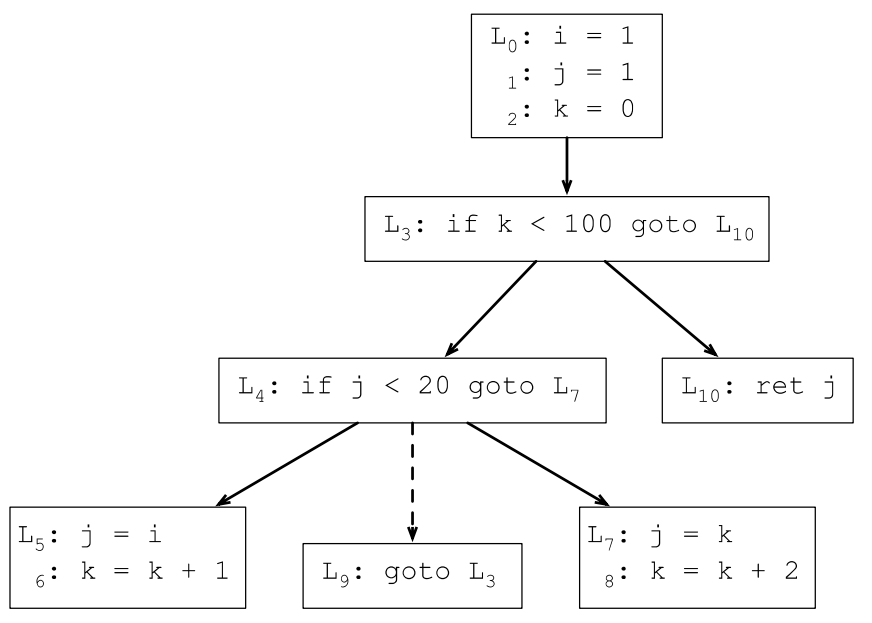### 7.3.4 計算支配前沿

DF(7) = {9}, DF(9) = {3}, DF(5) = {9}, DF(10) = {}

DF(4) = {3}

DF(3) = {3}

DF(0) = {}

### 7.3.5 插入φ函數### 7.3.7 變數重命名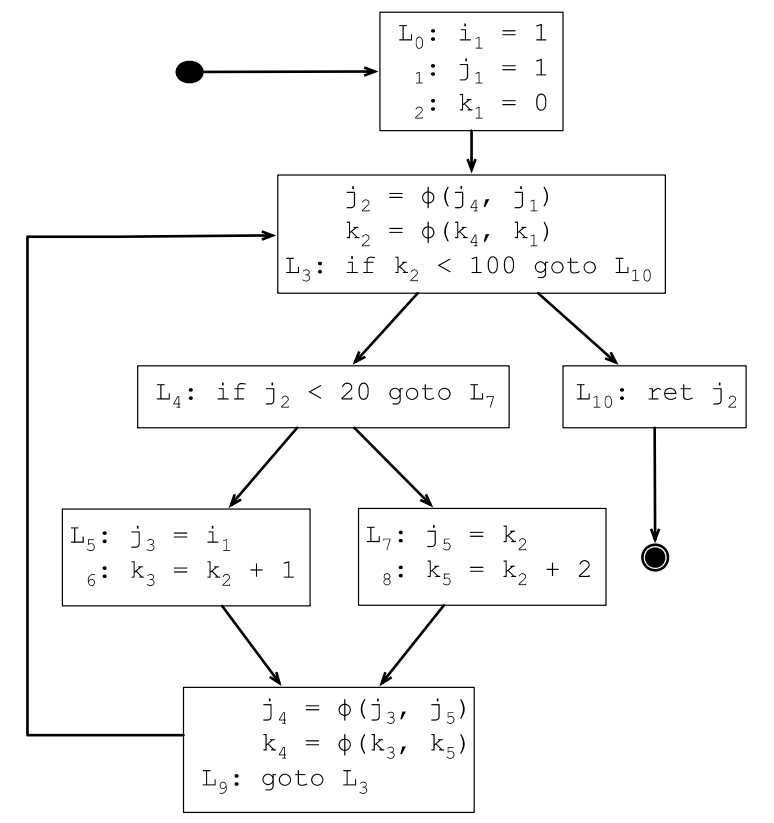### 7.3.8 優化SSA範式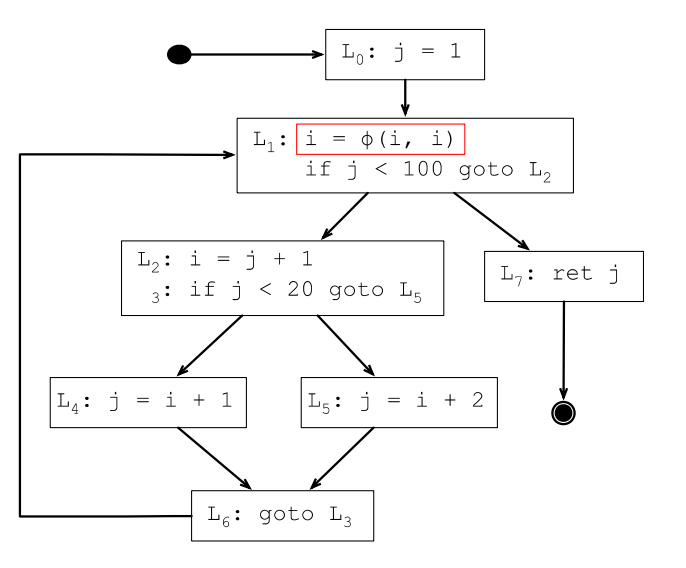## 7.4 使用SSA簡化分析

SSA範式可以用來簡化各種基於數據流的分析。SSA範式之前，數據流分析的某個變數的定義是一個集合，SSA範式轉換之後這些變數都變成了唯一定義；而且由於每個變數只有一次定義，相當於說每個變數都可以轉換成常量（迴圈內定義的變數除外，每次迴圈迭代，變數都會被重新定義）。

### 7.4.2 簡化常量傳播### 7.4.3 SSA範式轉換之後的生命周期分析

``` 1 For each statement S in the program:
2   IN[S] = OUT[S] = {}
3 For each variable v in the program:
4   For each statement S that uses v:
5     live(S, v)
6 live(S, v):
7   IN[S] = IN[S] ∪ {v}
8   For each P in pred(S):
9     OUT[P] = OUT[P] ∪ {v}
10     if P does not define v
11       live(P, v)```

## 7.5 SSA簡史

1. “An Efficient Method of Computing Static Single Assignment Form, ” appeared in the conference Record of the 16th ACM Symposium on principles of Programming Languages (Jan. 1989). https://c9x.me/compile/bib/ssa.pdf
2. Efficiently Computing Static Single Assignment Form and the Control Dependence Graph, ACM Transact~ons on Programmmg Languages and Systems, VO1 13, NO 4, October, le91, Pages 451.490. Efficiently computing static single assignment form and the control dependence graph (utexas.edu)
3. Lengauer, T. and Tarjan, R. "A Fast Algorithm for Finding Dominators in a Flowgraph", TOPLAS, 1:1 (1979) pp 121-141
4. Briggs, P. and Cooper, K. and Harvey, J. and Simpson, L. "Practical Improvements to the Construction and Destruction of Static Single Assignment Form", SP&E (28:8), (1998) pp 859-881• 目錄 一、前景回顧 二、規劃頁表 三、實現頁表 四、運行測試 一、前景回顧 前面我們已經介紹了分頁機制的運行原理，那麼如何開啟分頁機制呢，也簡單，分為如下三個步驟： 1、創建頁目錄表並初始化頁記憶體。 2、將頁目錄表地址賦值為CR3。 3、打開CR0寄存器的PG位。 可以看出頁表是分頁機制的核心，接下 ...
• 一、CDN是什麼？ CDN的全稱是Content Delivery Network，即內容分髮網絡。其目的是通過在現有的Internet中增加一層新的CACHE(緩存)層，將網站的內容發佈到最接近用戶的網路”邊緣“的節點，使用戶可以就近取得所需的內容（就近原則），提高用戶訪問網站的響應速度。從技術上 ...
• 分享嘉賓：葉聰 騰訊 技術專家 編輯整理：張智躍 內容來源：DataFun AI Talk「智能技術前沿實踐分享」 出品社區：DataFun 導讀： 本次分享系統介紹電腦視覺的基礎知識，如何利用這些識別演算法實現一個應用，同時進行部署、推廣這一整套流程。主要包括以下六個部分： 1、朋友圈爆款活動背後 ...
• 6月15日，由中國信通院主辦的以 “原生聚力，雲數賦能”為主題的“2022雲原生產業大會”在北京舉行。憑藉創新技術和領先實踐，騰訊云云巢榮獲“雲原生技術創新案例”獎。 騰訊云云巢是騰訊雲自主研發的一站式雲原生有狀態服務平臺，基於Kubernetes容器化架構，為各類有狀態服務提供統一的集群管理、資源 ...
• 一、開發背景 產品出設計稿要求做一個仿原生app簡訊驗證碼組件，花了兩小時搞出來一個還可以的組件，支持屏幕自適應，可以用於彈出框，或自己封裝的vue組件里，希望可以幫助那些被產品壓榨的同學，哈哈。😄 其核心思想就是利用一個輸入框使用css3,translate屬性，每輸入一次後向右位移一個單位位置 ...
• 認識WEB **「網頁」**主要是由文字、圖像和超鏈接等元素構成，當然除了這些元素，網頁中還可以包括音頻、視頻以及Flash等。 **「瀏覽器」**是網頁顯示、運行的平臺。 「瀏覽器內核」(排版引擎、解釋引擎、渲染引擎) 常見的瀏覽器及其內核 瀏覽器 內核 備註 IE Trident IE、獵豹安全 ...
• HTML各知識點總結： 基本標簽 標題標簽、段落標簽、換行標簽、水平線標簽、字體樣式標簽、註釋和特殊符號 網頁插入 圖像、超鏈接，視頻、音頻、列表、表格、表單、內聯框架等 超鏈接 錨鏈接、功能性鏈接 列表 有序列表、無序列表、自定義列表 表格 行、列、跨行、跨列 表單 提交格式、文本框、密碼框、單選 ...
• 昨天太晚就沒來得及更新，今天是spu管理界面，這個界面一共有三個界面需要切換，完成了兩個界面，而且今天的難度在於最後兩個章節，富有一定的邏輯性，當然中間也有很多需要註意的，比如ElementUI的照片牆需要添加list屬性而且值為你的數據並且必須是一個數組必須有name、url屬性 一.spu管理 ...